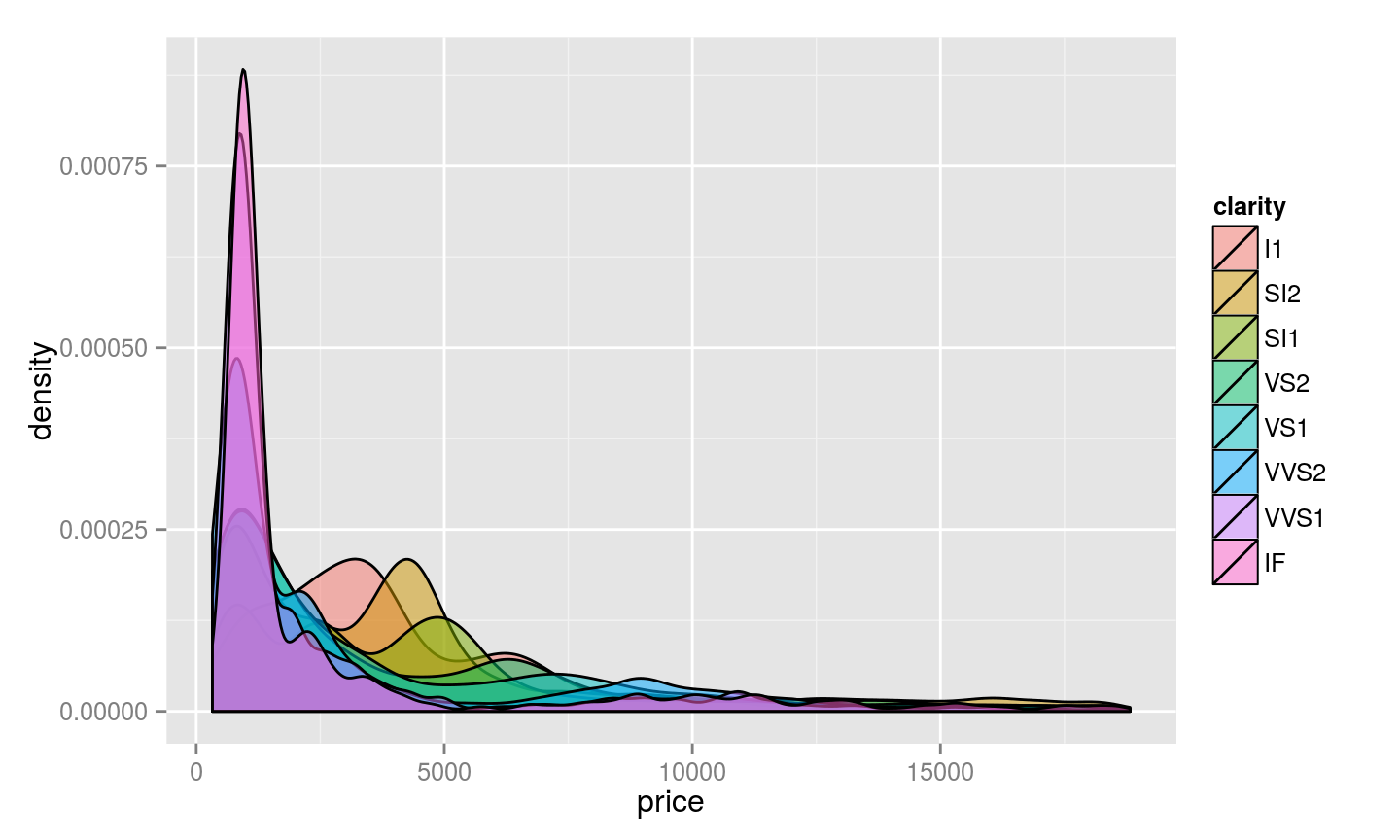Curated Data Sets

Here are two data sets of particular note:

Goal: Ask a question, answer it with a picture!

Some ideas:

• What is the distribution of gene or protein sizes across the yeast genome? Of introns? Of functional RNAs?
• Are there correlations between the processes proteins are involved in and their size? Or location within the genome?
• Are there biases in which genes contain introns?
• Do short chromosomes have more or longer introns? [credit to Joseph]

Grammar of Graphics in R (ggplot2)

library(ggplot2)

Constructing a plot

# Here's the structure of the diamonds dataset
##   carat       cut color clarity depth table price    x    y    z
## 1  0.23     Ideal     E     SI2  61.5    55   326 3.95 3.98 2.43
## 2  0.21   Premium     E     SI1  59.8    61   326 3.89 3.84 2.31
## 3  0.23      Good     E     VS1  56.9    65   327 4.05 4.07 2.31
## 4  0.29   Premium     I     VS2  62.4    58   334 4.20 4.23 2.63
## 5  0.31      Good     J     SI2  63.3    58   335 4.34 4.35 2.75
## 6  0.24 Very Good     J    VVS2  62.8    57   336 3.94 3.96 2.48
# Construct a ggplot object to visualize the relationship between clarity and depth
p <- ggplot(diamonds, aes(clarity, depth))
p + geom_point()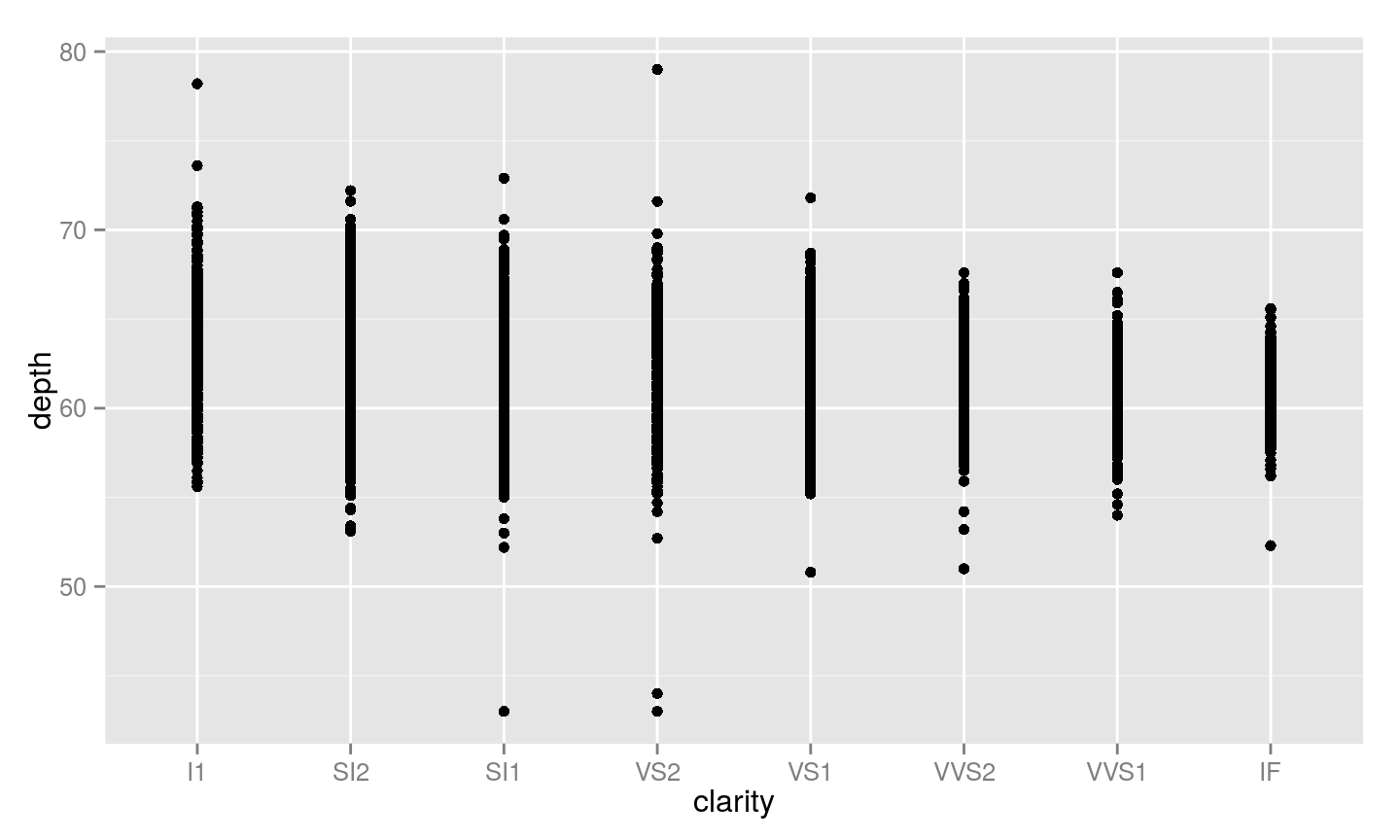p + geom_boxplot()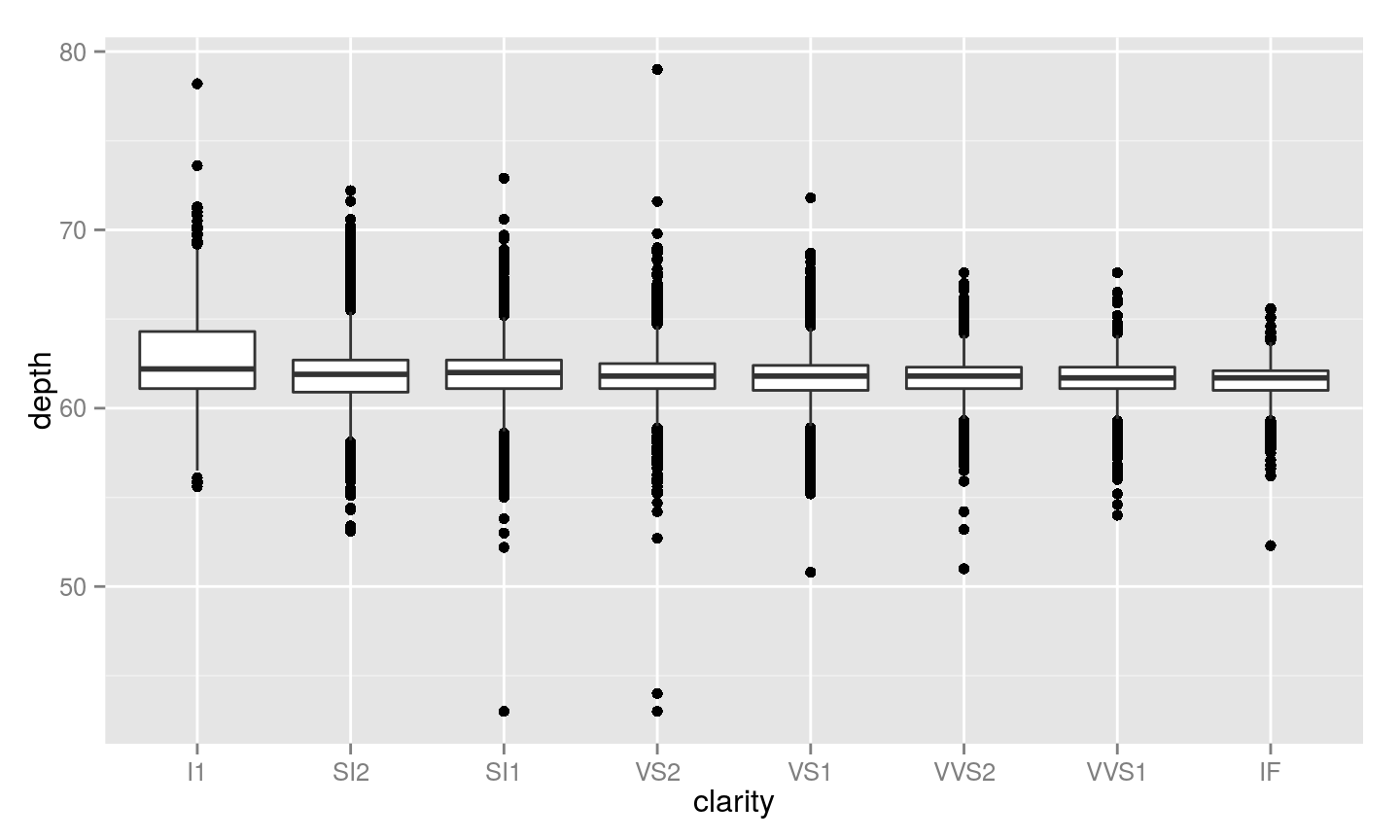Single variable distributions

# Visualize the distribution of the depth variable
p2 <- ggplot(diamonds, aes(depth))
# Histogram
p2 + geom_histogram()
## stat_bin: binwidth defaulted to range/30. Use 'binwidth = x' to adjust this.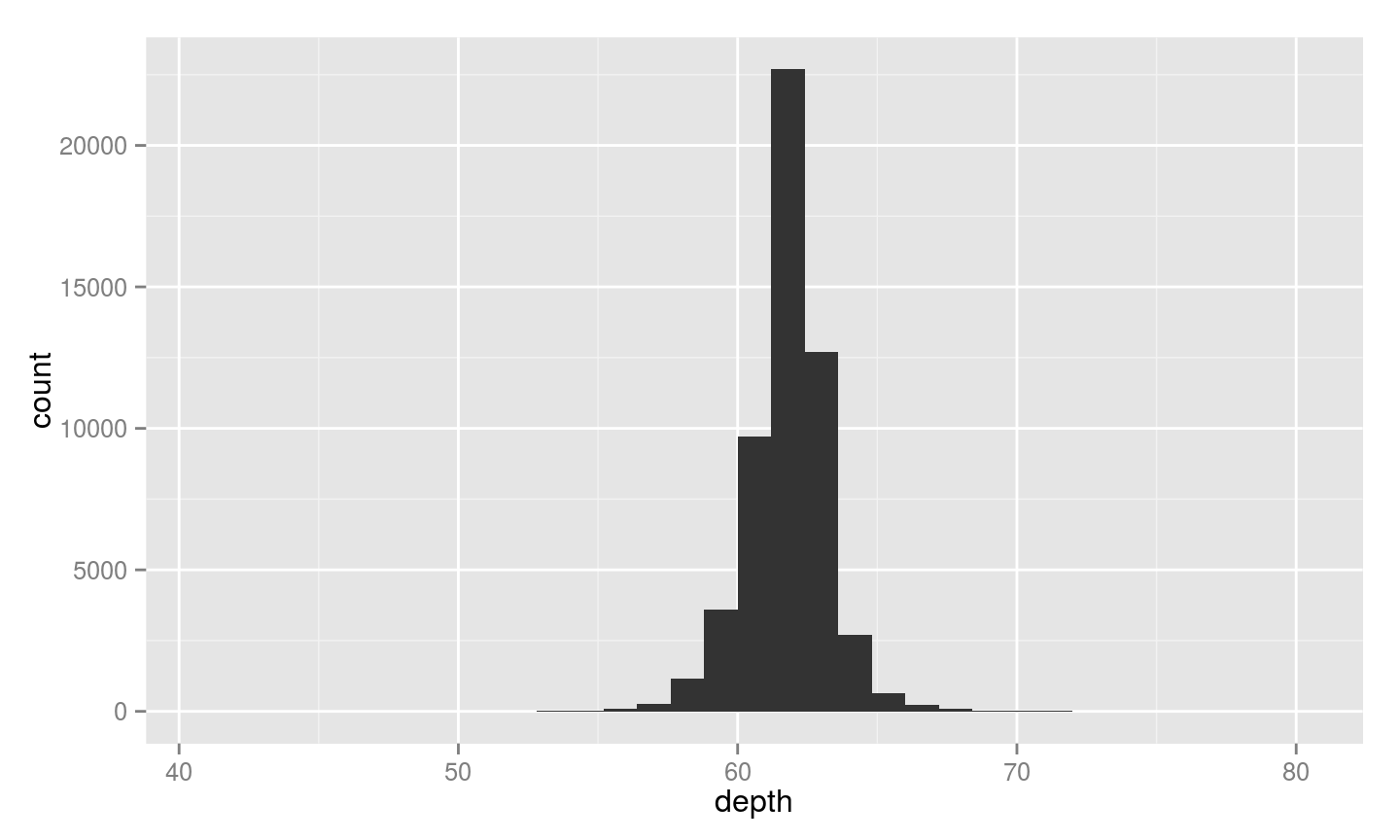# Density distribution
p2 + geom_density()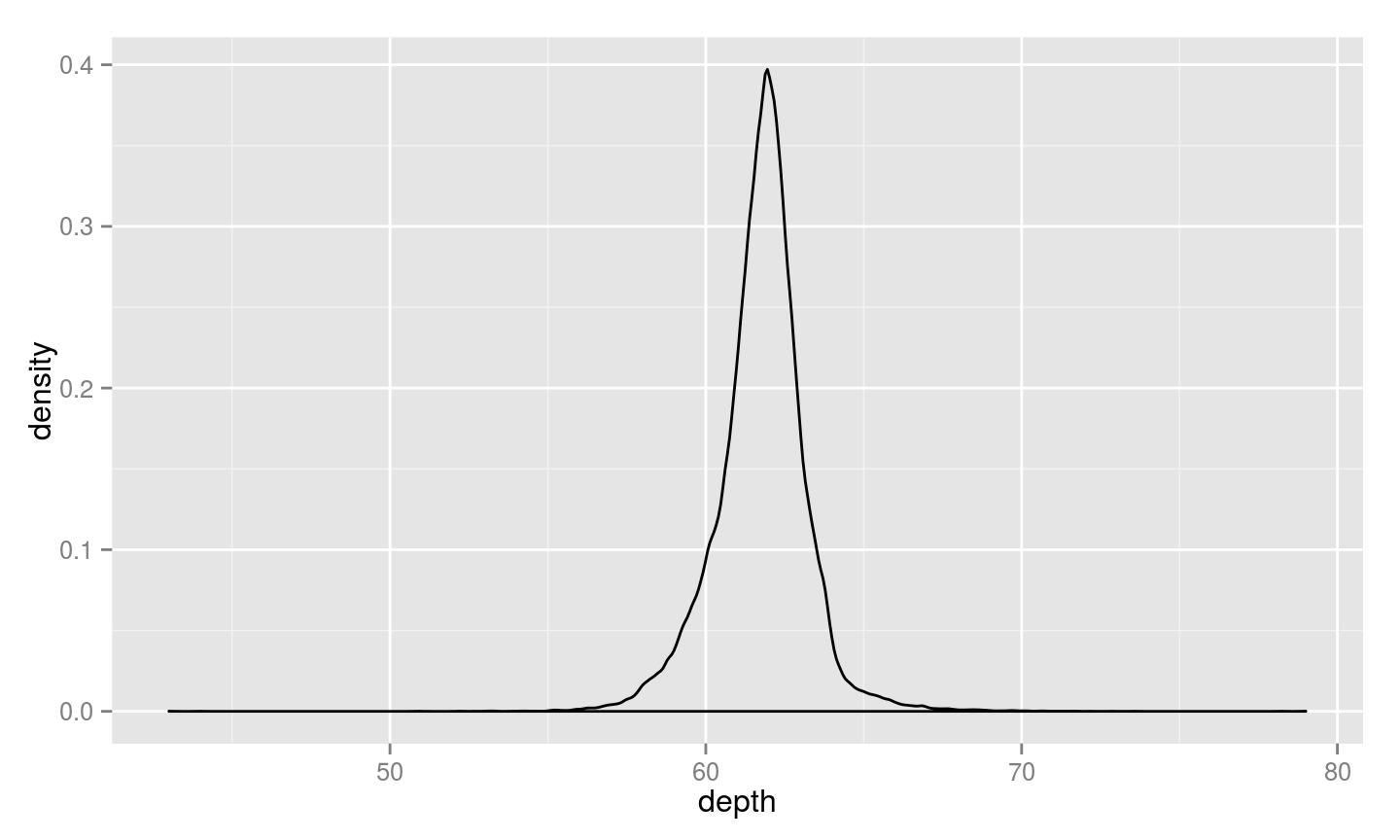Setting axis limits

# Set x-axis limits
p2 + geom_density() + xlim( c(55, 65) )
## Warning: Removed 857 rows containing non-finite values (stat_density).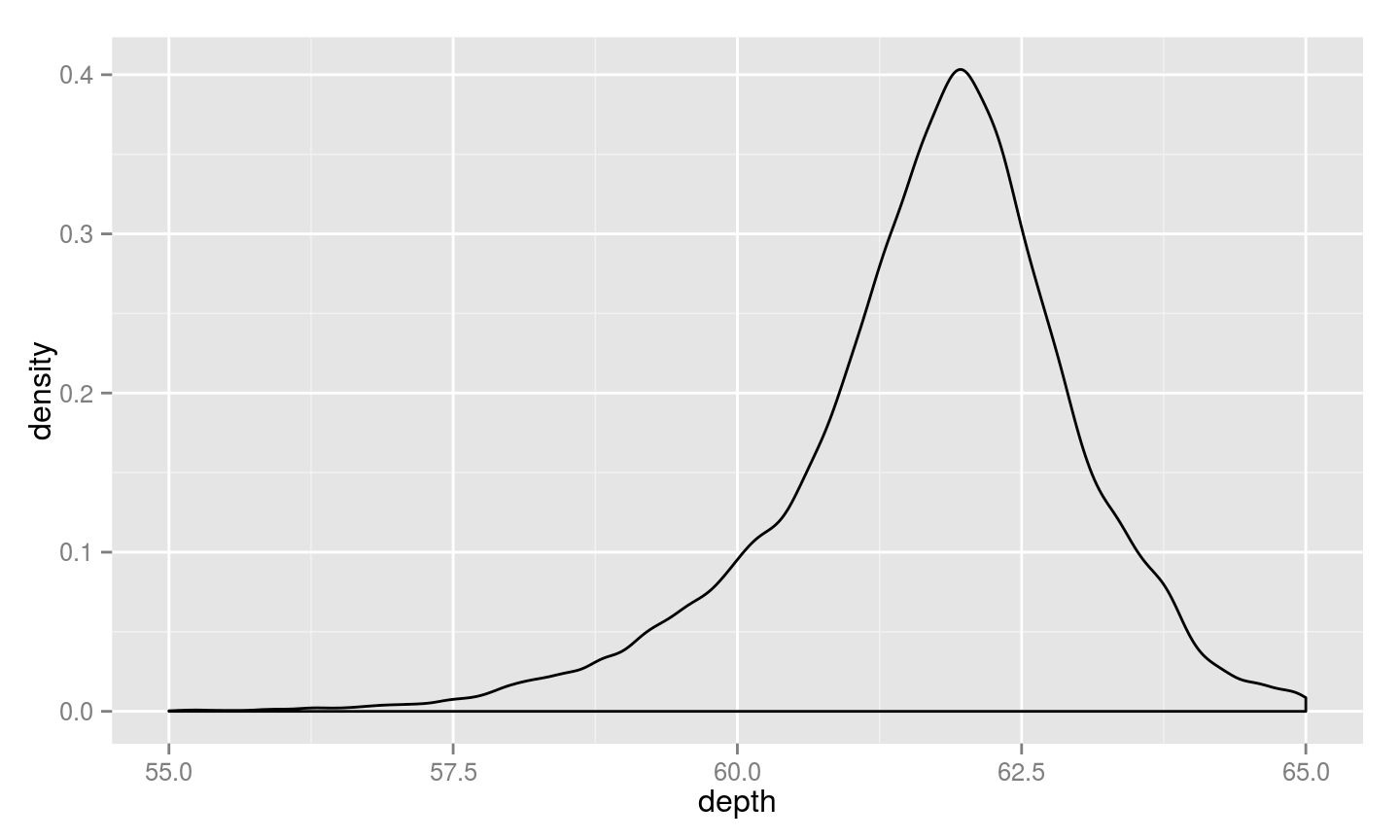Two quantitative variables

# Visualize depth as a function of price
p3 <- ggplot( diamonds, aes(price, depth) )
# Use points (scatter plot)
p3 + geom_point()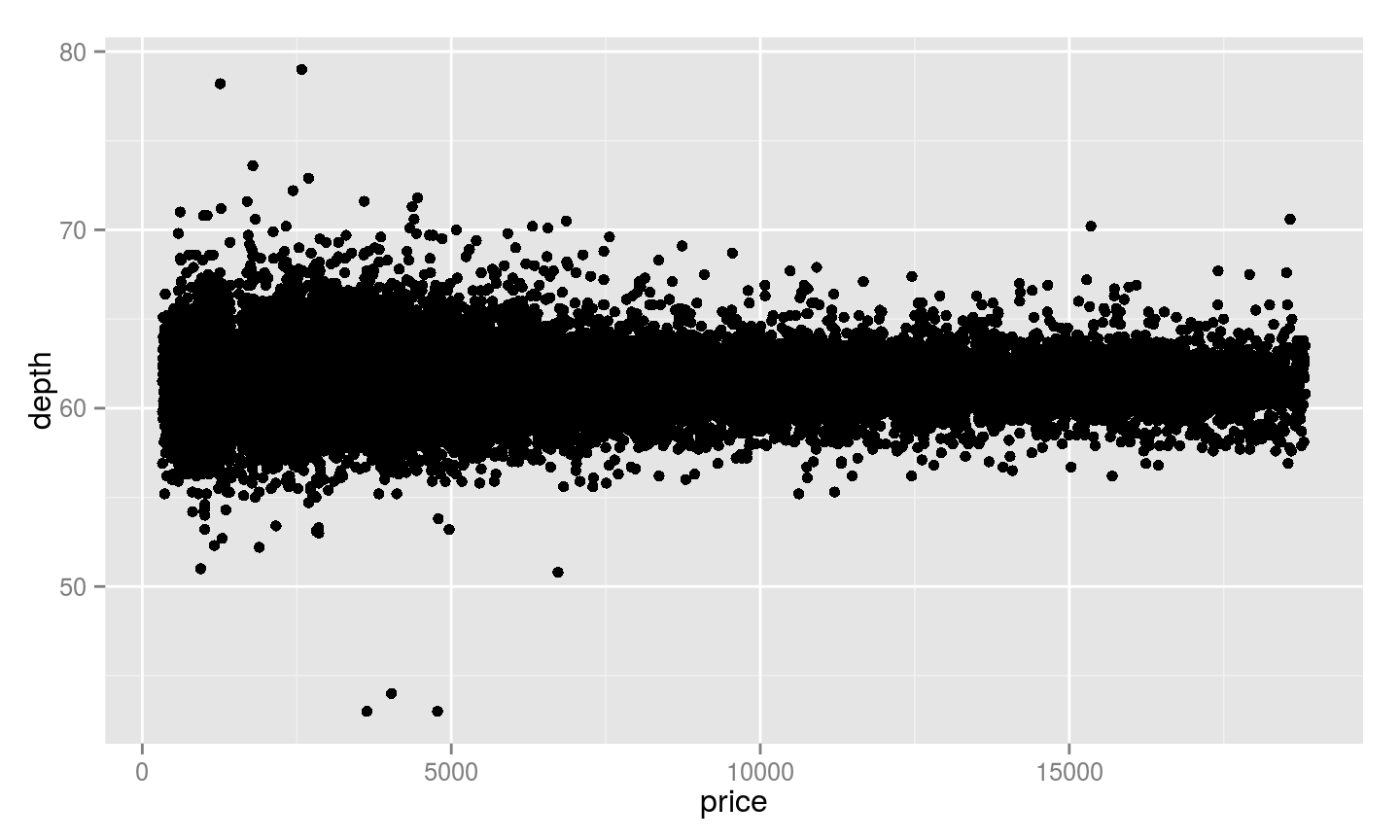Data grouping and secondary visualizations

# Visualize depth as a function of price; use color to visualize clarity label
p4 <- ggplot( diamonds, aes(price, depth, color = clarity) )
# Use points (scatter plot)
p4 + geom_point()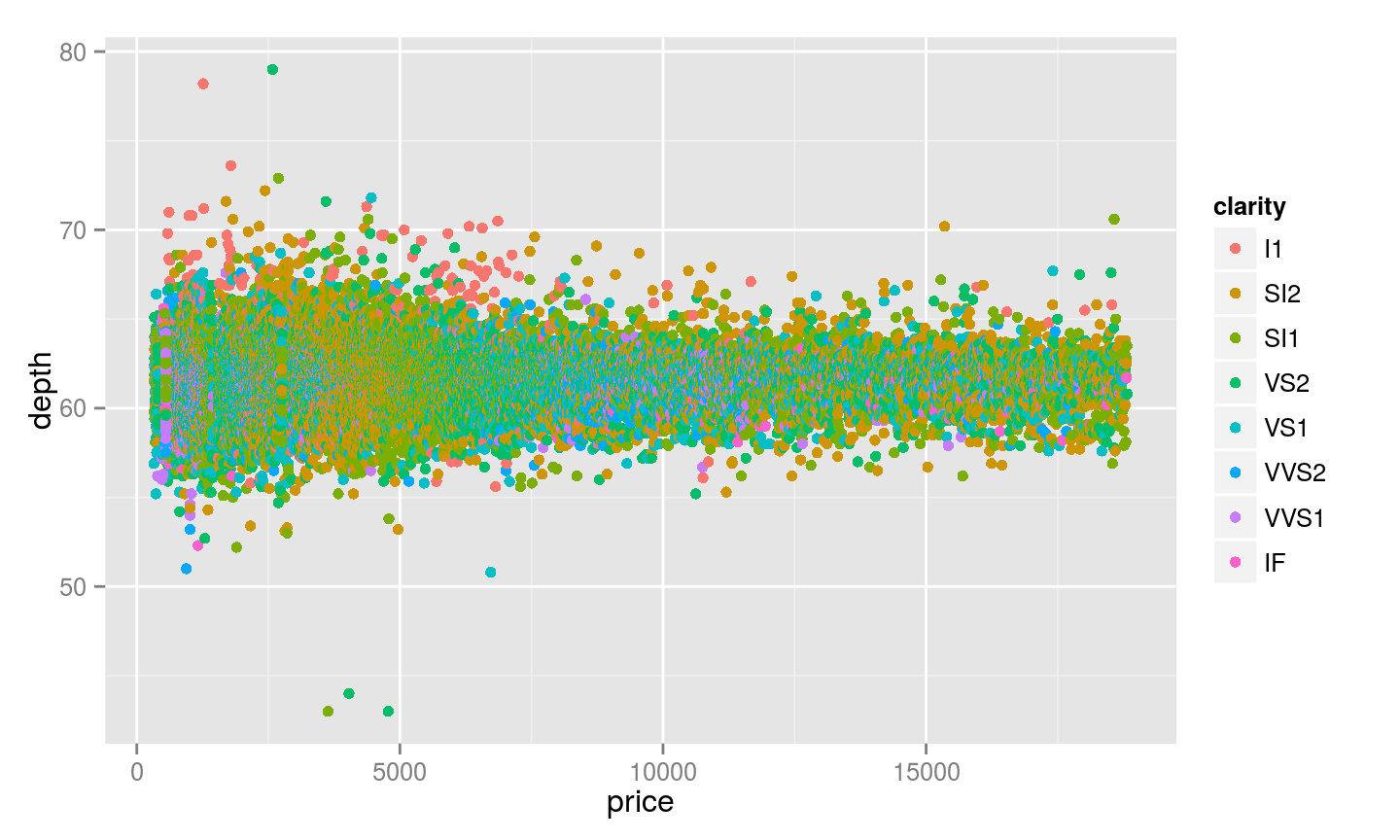For visualizations that use solid objects, you can also specify a fill argument:

p5 <- ggplot(diamonds, aes( price, fill = clarity) )
# Setting the alpha channel to 50% makes the density plots transparent
p5 + geom_density(alpha = 0.5)